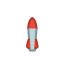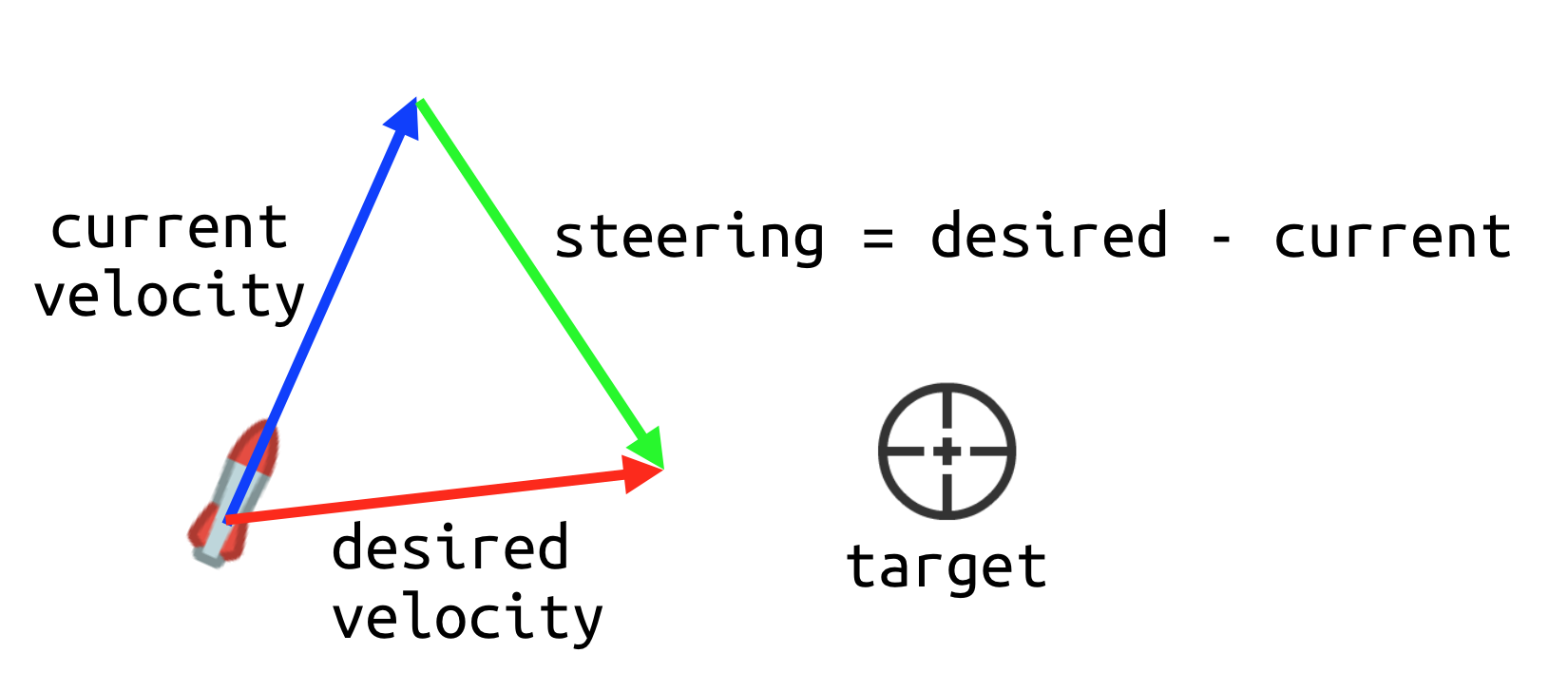# Homing missile

## Problem

You need a “homing missile” - a projectile that will seek a moving target.

## Solution

For this example, we’ll use an `Area2D` node for the projectile. Areas are typically good choices for bullets because we need to detect when they contact something. If you also need a bullet that bounces/ricochets, one of the `PhysicsBody` type node might be a better choice.

The node setup and behavior of the missile is the same you would use for a “dumb” bullet. If you’re creating many bullet types, you can use inheritance to base all your projectiles on the same core setup.

The nodes we’ll use:

`````` Area2D: Missile
Sprite
CollisionShape2D
``````

For the texture, you can use any image you like. Here’s an example one:Set up the nodes and configure the sprite’s texture and the collision shape. Make sure to rotate the `Sprite` node by `90°` so that it’s pointing to the right, ensuring it matches the parent’s “forward” direction.

Add a script and connect the `Area2D`’s `body_entered` signal and the `Timer`’s `timeout` signal.

Here’s the starting script:

``````extends Area2D

export var speed = 350

var velocity = Vector2.ZERO
var acceleration = Vector2.ZERO

func start(_transform):
global_transform = _transform
velocity = transform.x * speed

func _physics_process(delta):
velocity += acceleration * delta
velocity = velocity.clamped(speed)
rotation = velocity.angle()
position += velocity * delta

func _on_Missile_body_entered(body):
queue_free()

queue_free()
``````

This creates a “dumb” bullet that travels in a straight line when fired. To use this projectile, instance it and call its `start()` method with the desired `Transform2D` to set its position and direction.

See the related recipes section below for more information.

To change the behavior to seek a target, we’ll use the `acceleration`. However, we don’t want the missile to “turn on a dime”, so we’ll add a variable to control its “steering” force. This will give the missile a turning radius that can be adjusted for different behavior. We also need a `target` variable so that the missile knows what to chase. We’ll set that in `start()` as well:

``````export var steer_force = 50.0

var target = null

func start(_transform, _target):
# add this line:
target = _target
``````

To change the missile’s direction to move toward the target, it needs to accelerate in that direction (acceleration is change in velocity). The missile “wants” to move straight towards the target, but its current velocity is pointing in a different direction. Using a little vector math, we can find that difference:The green arrow represents the needed change in velocity (i.e. `acceleration`). However, if we turn instantly, that will look unnatural, so the “steering” vector’s length needs to be limited. This is the purpose of the `steer_force` variable.

This is the function to calculate that acceleration. Note that if there’s no target, there will be no steering, so the missile remains traveling in a straight line.

``````func seek():
var steer = Vector2.ZERO
if target:
var desired = (target.position - position).normalized() * speed
steer = (desired - velocity).normalized() * steer_force
return steer
``````

Finally, the resulting steer force must be applied in `_physics_process()`:

``````func _physics_process(delta):
acceleration += seek()
velocity += acceleration * delta
velocity = velocity.clamped(speed)
rotation = velocity.angle()
position += velocity * delta
``````

Here’s an example of the results, with a little extra visual flair such as particle smoke and explosions:

Here’s the full script, including the above effects. See related recipes for details.

``````extends Area2D

export var speed = 350
export var steer_force = 50.0

var velocity = Vector2.ZERO
var acceleration = Vector2.ZERO
var target = null

func start(_transform, _target):
global_transform = _transform
rotation += rand_range(-0.09, 0.09)
velocity = transform.x * speed
target = _target

func seek():
var steer = Vector2.ZERO
if target:
var desired = (target.position - position).normalized() * speed
steer = (desired - velocity).normalized() * steer_force
return steer

func _physics_process(delta):
acceleration += seek()
velocity += acceleration * delta
velocity = velocity.clamped(speed)
rotation = velocity.angle()
position += velocity * delta

func _on_Missile_body_entered(body):
explode()